Download Presentation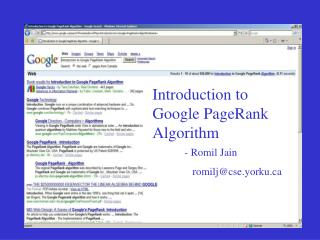- Romil Jain romilj@cse.yorku

# - Romil Jain romilj@cse.yorku - PowerPoint PPT Presentation

Introduction to Google PageRank Algorithm. - Romil Jain romilj@cse.yorku.ca. World Wide Web. WWW is HUGE. Approximate estimations : ~50 million active web sites ~25 billion web pages ~1 billion users. There are a large number of search engines too :I am the owner, or an agent authorized to act on behalf of the owner, of the copyrighted work described.
Download Presentation## - Romil Jain romilj@cse.yorku

An Image/Link below is provided (as is) to download presentation

Download Policy: Content on the Website is provided to you AS IS for your information and personal use and may not be sold / licensed / shared on other websites without getting consent from its author.While downloading, if for some reason you are not able to download a presentation, the publisher may have deleted the file from their server.

- - - - - - - - - - - - - - - - - - - - - - - - - - E N D - - - - - - - - - - - - - - - - - - - - - - - - - -
Presentation TranscriptIntroduction to Google PageRank Algorithm

- Romil Jain

romilj@cse.yorku.caWorld Wide Web
• WWW is HUGE. Approximate estimations :
• ~50 million active web sites
• ~25 billion web pages
• ~1 billion users
• There are a large number of search engines too :
• At least 3,105 search engines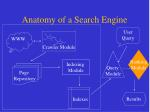Crawler Module

Ranking

Module

Query

Module

Indexing

Module

Page

Repository

Indexes

Results

Anatomy of a Search Engine

User Query

WWWRanking Module
• Key is to find those pages that the user desires
• Takes a set of relevant web pages and ranks them
• Rank is generally a function of:
• Content Score &
• Popularity Score (The focus of this talk)
• E.g. “What are some good Indian restaurants in Toronto?”r(Pj)

r(Pi) : PageRank of page Pi

Bi : set of pages pointing to Pi

| Pj | : # out-links from Pj

r(Pi) =

|Pj|

Pj  Bi

Ranking Web Pages by Popularity
• PageRank algorithm, given by Sergey Brin and Larry Page in 1998 
• Exploits the linked structure of the web for computing popularity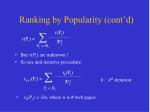k : kth iteration

rk(Pj)

rk+1(Pi) =

|Pj|

Pj  Bi

Ranking by Popularity (cont’d)

r(Pj)

• But r(Pj) are unknown !
• So use and iterative procedure:

r(Pi) =

|Pj|

Pj  Bi

• r0(Pj) = 1/n, where n is # web pages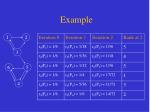Example

1

2

3

6

5

4P1

P2

P3

P4

P5

P6

P1

0

1/2

1/2

0

0

0

P2

0

0

0

0

0

0

Hyperlink Matrix H =

P3

1/3

1/3

0

0

1/3

0

P4

0

0

0

0

1/2

1/2

P5

0

0

0

1/2

0

1/2

P6

0

0

0

1

0

0

rk(Pj)

rk+1(Pi) =

|Pj|

Pj  Bi

r0(Pj) = 1/n

Matrix Notation

1

2

3

6

5

4

(k+1)T = (k)TH,

 (k)T : PageRank vector after kth iteration

 (0)T : 1/n eT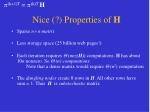(k+1)T = (k)T H

Nice (?) Properties of H
• Sparse n n matrix
• Less storage space (25 billion web pages!)
• Each iteration requires  (nnz(H)) computations. H has about 10n nonzero. So  (n) computations.
• Note that a dense matrix would require  (n2) computation
• The dangling nodescreate 0 rows in H. All other rows have sum = 1. Thus H is substochastic matrix(k+1)T = (k)T H

1

2

with (0)T = (1 0),(k)T will flip-flop between (1 0) and (0 1) !

Issues with Iterative Process
• Will it converge or continue indefinitely?
• What properties of Hwill ensure convergence?
• Does convergence depend on (0)T ?
• How long will it take to converge i.e. what k is the fixed point?
• Does a converged T give useful page ranks?

All these questions can be answered using theory of

Markov Chains & Stochastic Matrices…Stochastic Matrix

A stochastic matrix S is:

• n n matrix with each row-sum = 1
• for each sij ,0  sij1

Markov Chain for a Random Surfer

Transition Probability MatrixPower of Stochastic Matrix

If we start from C, what is the probability that we will reach B in 2 steps?

P(CB2) = P(CA)P(AB) + P(CB)P(BB) + P(CC)P(CB)It can be proven for a stochastic

matrix S that:

lim Sn = S* , if 0  sij  1

n

Power Convergence

In 3, 4, 5, 6, 7 steps?State Vector Transition

If xTis a stochastic probability distribution vector of a given state, then:

x (k+1)T= x (k)TS

Similar to (k+1)T = (k)T H, except that His not stochastic!x (n+1)T = x (n)T S

State Vector Convergence

lim x(n)T = x (0)T lim Sn = x (0)TS* = x*

n nP1

P2

P3

P4

P5

P6

P1

0

1/2

1/2

0

0

0

P2

0

0

0

0

0

0

Hyperlink Matrix H =

P3

1/3

1/3

0

0

1/3

0

P4

0

0

0

0

1/2

1/2

P5

0

0

0

1/2

0

1/2

P6

0

0

0

1

0

0

The problem is due to these dangling rows

H is not stochastic!

(k+1)T = (k)T HP1

P2

P3

P4

P5

P6

P1

0

1/2

1/2

0

0

0

P2

1/6

1/6

1/6

1/6

1/6

1/6

P3

1/3

1/3

0

0

1/3

0

P4

0

0

0

0

1/2

1/2

P5

0

0

0

1/2

0

1/2

P6

0

0

0

1

0

0

Dangling rows

eliminated…

S =

Adjustment 1 to H

A random surfer can randomly “jump” to any page after he encounters a dangling node

S = H + a(1/n eT)

a is called the dangling node vector. ai = 1 if page i is dangling otherwise 0.G = S + (1 - ) E , 0    1

E = 1/n eeT is called the teleportation matrix

 is the % of time a user surfs or teleports

G is called the Google Matrix

(k+1)T = (k)T S

Adjustment 2 to H

0  sij  1 not true for S!

A random surfer can randomly “teleport” to any page irrespective of the current page.Finally we have G!

G = S + (1 - ) E , 0    1

(k+1)T = (k)T G

• Gis stochastic
• 0  gij  1 true for G

Therefore the above equation converges for any (0)T

But now G is no longer sparse . In fact it is completely dense!(k+1)T = (k)T G

Fortunately…

G = S + (1 - ) E

= S + (1 - ) 1/n eeT

= (H + 1/n aeT) + (1 - ) 1/n eeT

= H + (a + (1 - ) e) 1/n eT

Therefore:

(k+1)T = (k)T G

=  (k)T H + ( (k)T a + (1 - ) (k)T e ) 1/n eT

=  (k)T H + ( (k)T a + (1 - )) 1/n eT (?)

Now vector multiplications are done on extremely sparse H(k+1)T = (k)T G

Importance of 

G = S + (1 - ) E , 0    1

(k+1)T = (k)T G

What  must be chosen?

It can be shown that rate of convergence is the rate at which

k  0

  0, T converges immediately, but completely unrealistic!

  1, Tmay never converge, again unrealistic !

We want  to be as close as possible to 1(k+1)T = (k)T G

 = 0.85 Saves the Day

G = S + (1 - ) E, 0    1

Brin & Page initially chose  = 0.85, and this is still the value

Takes about 50 iterations (3 days) to converge sufficiently

Accuracy is 50= .8550 .000296, which is sufficient for(k+1)T = (k)T G

Importance of Teleportation Matrix E

G = S + (1 - ) E

Initially we had E = 1/n eeT

This means that a random surfer can teleport to any web page with equal probability 1/n

Instead of 1/n eeT use evT , where vTis the

personalization or teleportation vector.

vT is used to counter-act link farms (like SearchKing.com)(k+1)T = (k)T G

Issue: Sensitivity of PageRank

It can be shown that:

1

d (k)T

d 

1 - 

as   1, 1/(1- ) 

So, PageRank is quite sensitive to small changes in the web.

Google computes PageRank from scratch every month!

Can we compute i+1 from i without computing i+1 from scratch?(k+1)T = (k)T G

Issue: PageRank is Query Independent!
• PageRank is pre-computed.
• It means that to be better linked is more important than to contain the search terms
• This is significant because a badly linked page, might be popular within the community of pages with the same topic

A rosy idea: Is it feasible to compute PageRank after the relevant

documents have been retrieved?(k+1)T = (k)T G

Issue: PageRank is Dead!

Not for now, but is susceptible to a lot of damage:

• PageRank is based upon an ideal democratic structure of the web
• But hackers, spammers and SEO’s know too much about Google to skew the rankings
• Typical examples are Link Farms and Google Bombs.
• Bloggers created a bomb where if you typed “miserable failure” then Google would take you to www.whitehouse.gov!

How can we detect and fight Rank Skewing?References
• The size of the World Wide Web, May 2007.

http://www.pandia.com/sew/383-web-size.html.

• Search Engines Worldwide, Jan 2003. http://home.inter.net/takakuwa/search/search.html .
• Langville and Meyer. Google’s PageRank and Beyond. Princeton University Press, 2006.
• Brin and Page. The Anatomy of a Large-scale Hypertextual Web Search Engine. Computer Networks and ISDN Systems, 1998.